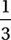# SAT Math Multiple Choice Question 674: Answer and Explanation

### Test Information

Question: 674

14. A culture of bacteria initially contained p cells, where p > 100. After one hour, this population decreased by. In the second and third hours, however, the population increased by 40% and 50%, respectively. At the end of those first three hours, what was the population of the culture?

• A. 1.3p
• B. 1.4p
• C. 1.5p
• D. 1.6p

We might begin by plugging in a number for p. Let's say p = 120 cells to start. We are told that after one hour the population decreased by. Sinceof 120 is 40, the population decreased by 40 and the population was then 120 - 40 = 80 cells. In the second hour, the population increased by 40%. Increasing a number by 40% is equivalent to it by 1.40 (because it becomes 140% of what it was), so the population was then 80(1.40) = 112 cells. In the third hour, the population increased by 50%, so it became 112(1.50) = 168 cells.